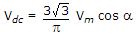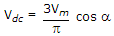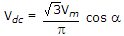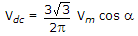# Electronics and Communication Engineering - Power Electronics

### Exercise :: Power Electronics - Section 4

11.

A 3 phase fully controlled bridge converter is fed by a 3 phase system having phase voltage v = Vm sin ωt. The firing angle is a. The dc output voltage is

 A.B.C.D.Explanation:

No answer description available for this question. Let us discuss.

12.

Which of these commutation methods uses an external pulse for commutation?

 A. Class A B. Class C C. Class E D. Class F

Explanation:

No answer description available for this question. Let us discuss.

13.

In a single phase full wave regulator feeding an R-L load, the power factor angle of load is θ. The range of control of firing angle a is

 A. 0 ≤ a ≤ p B. θ ≤ a ≤ p C. 0 ≤ a ≤ (p - θ) D. θ ≤ a ≤ (p + θ)

Explanation:

No answer description available for this question. Let us discuss.

14.

A separately excited dc motor is run from a dc source through a chopper. At 600 rpm and rated torque, the duty cycle of chopper is 0.8. At 600 rpm and half the rated torque, the duty cycle is likely to be

 A. 0.9 B. 0.8 C. 0.75 D. 0.6

Explanation:

No answer description available for this question. Let us discuss.

15.

A relaxation oscillator uses

 A. tunnel diode B. UJT C. both tunnel diode and UJT D. PIN diode